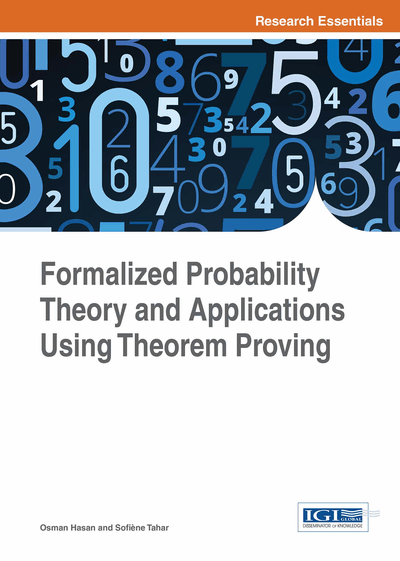# Information Measures

DOI: 10.4018/978-1-4666-8315-0.ch009
Available
\$37.50
No Current Special Offers

## Abstract

This chapter presents a higher-order-logic formalization of the main concepts of information theory (Cover & Thomas, 1991), such as the Shannon entropy and mutual information, using the formalization of the foundational theories of measure, Lebesgue integration, and probability. The main results of the chapter include the formalizations of the Radon-Nikodym derivative and the Kullback-Leibler (KL) divergence (Coble, 2010). The latter provides a unified framework based on which most of the commonly used measures of information can be defined. The chapter then provides the general definitions that are valid for both discrete and continuous cases and then proves the corresponding reduced expressions where the measures considered are absolutely continuous over finite spaces.
Chapter Preview
Top

## 9.1 Formalization Of Radon-Nikodym Derivative

The Radon-Nikodym derivative of a measure v with respect to the measure u is defined as a non-negative measurable function f, satisfying the following formula, for any measurable set A.

(9.1)

The Radon-Nikodym derivative is formalized in HOL4 as (Mhamdi, 2013):

• Definition 9.1

```Ⱶ RN_deriv m v =
@f. f IN measurable (X,S) Borel ɅxX, 0f x ɅaS, integral m (x. f xIa x) = v a```

where @ denotes the Hilbert-choice operator. The existence of the Radon-Nikodym derivative is guaranteed for absolutely continuous measures by the Radon-Nikodym theorem stating that if v is absolutely continuous with respect to µ, then there exists a non-negative measurable function f such that for any measurable set A,

(9.2)

The Radon-Nikodym theorem can be verified in HOL4 for finite measures, which can be easily generalized to σ-finite measures.

• Theorem 9.1

```Ⱶm v s st.
measure_space (s, st, m) ˄
measure_space (s, st, v) ˄
abs_cont (s, st, m) (s, st, v)f. fmeasurable (s, st) Borel ˄xs, 0f x < ∞˄ast, integral m (x. f xIa  x) = v a```

## Complete Chapter List

Search this Book:
Reset